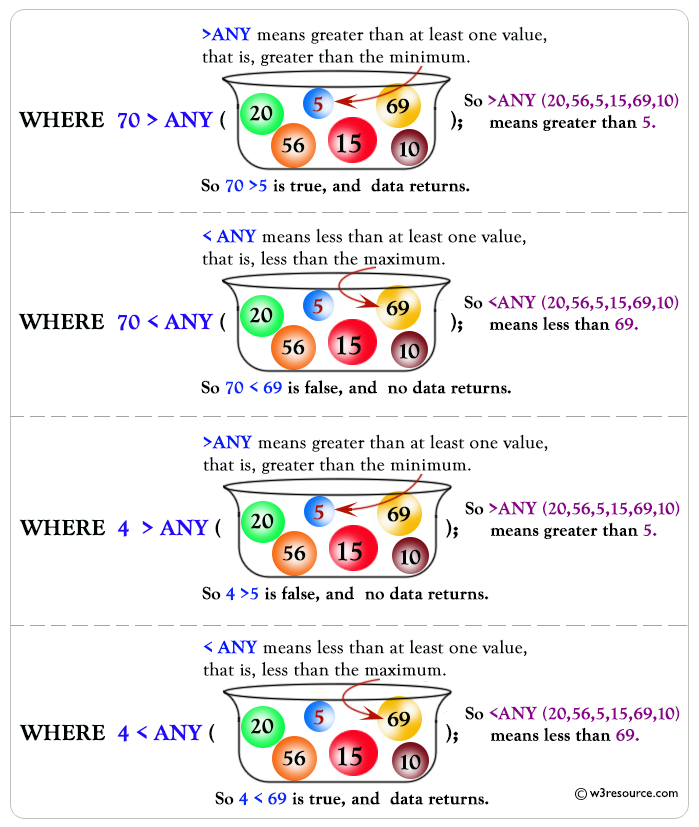# SQL ANY Operator

## ANY Operator

ANY compares a value to each value in a list or results from a query and evaluates to true if the result of an inner query contains at least one row. ANY must be preceded by comparison operators. Suppose using greater than ( >) with ANY means greater than at least one value.

Syntax:

```SELECT [column_name... | expression1 ]
FROM [table_name]
WHERE expression2 comparison_operator {ALL | ANY | SOME} ( subquery )
```

Parameters:

Name Description
column_name Name of the column of the table.
expression1 Expression made up of a single constant, variable, scalar function, or column name and can also be the pieces of a SQL query that compare values against other values or perform arithmetic calculations.
table_name Name of the table.
WHERE expression2 Compares a scalar expression until a match is found for ANY operator. One or more rows must match the expression to return a Boolean TRUE value for the ANY operator.
comparison_operator Compares the expression to the subquery. The comparison must be a standard comparison operator (=, <>, !=, >, >=, <, or <=).

Pictorical Presentation: SQL ANY OperatorDBMS Support : SQL ANY Operator

 DBMS Command MySQL Supported PostgreSQL Supported SQL Server Supported Oracle Supported

Example: SQL ANY operator

To get 'agent_code', 'agent_name', 'working_area', 'commission' from 'agents' table with following conditions -

1. 'agent_code' should be any 'agent_code' from 'customer' table, which satisfies the condition bellow :

a) 'cust_country' in the 'customer' table must be 'UK',

the following SQL statement can be used :

``````SELECT agent_code,agent_name,working_area,commission
FROM  agents
WHERE agent_code=ANY(
SELECT agent_code FROM customer
WHERE cust_country='UK');``````

Sample table: customer

Sample table: agents

Output:

```AGENT_CODE AGENT_NAME           WORKING_AREA         COMMISSION
---------- -------------------- -------------------- ----------
A009       Benjamin             Hampshair                   .11
A003       Alex                 London                      .13
A006       McDen                London                      .15
```

SQL ANY operator in nested subquery

In this example, we have discussed how SQL ANY operator can work with nested select statement.

Sample table: customer

Sample table: agents

Sample table: orders

To get 'agent_code', 'agent_name', 'working_area', 'commission' from 'agents' table with following conditions -

1. 'agent_code' should be any 'agent_code' from 'customer' table, which satisfies the condition bellow :
a) 'agent_code' should be any 'agent_code' from 'orders' table, which satisfies the condition bellow :
i) 'advance_amount' of 'orders' table must be more than 600,

the following SQL statement can be used :

``````SELECT agent_code,agent_name,working_area,commission
FROM  agents
WHERE agent_code=ANY(
SELECT agent_code FROM customer
WHERE agent_code =ANY
(SELECT agent_code FROM orders
``````

Output:

```AGENT_CODE AGENT_NAME           WORKING_AREA         COMMISSION
---------- -------------------- -------------------- ----------
A008       Alford               New York                    .12
A010       Santakumar           Chennai                     .14
A005       Anderson             Brisban                     .13
A002       Mukesh               Mumbai                      .11
```

SQL ANY operator with GROUP BY

In this example we have discussed the usage of SQL ANY operator with SQL GROUP BY in a select statement.

Sample table: customer

Sample table: agents

Sample table: orders

To get 'agent_code', and 'advance_amount' from 'orders' table with following conditions -

1. 'advance_amount' of 'orders' table must be more than 600,
2. 'agent_code' should be any 'agent_code' from 'customer' table, which satisfies the condition bellow :
a) 'agent_code' should be any 'agent_code' from 'agents' table, which satisfies the condition bellow :
i) 'commission' of 'agents' table must be more than or equal to .12,
3. 'agent_code' in 'orders' table must be in a group,

the following SQL statement can be used :

``````SELECT DISTINCT(agent_code),advance_amount
FROM orders
AND agent_code=ANY(
SELECT agent_code
FROM customer
WHERE agent_code=ANY(
SELECT agent_code FROM agents
WHERE commission>=.12
GROUP BY agent_code));
``````

Output:

```AGENT_CODE ADVANCE_AMOUNT
---------- --------------
A005                 1800
A008                 2000
A010                  800
A005                  700
A008                 1000
A010                  900
A010                  700
```

SQL ANY with group by and order by

In this example we have discussed the usage of SQL ANY operator with GROUP BY and ORDER BY clause in a select statement.

Sample table: customer

Sample table: agents

Sample table: orders

To get 'agent_code', and 'advance_amount' from 'orders' table with following conditions -

1. 'advance_amount' of 'orders' table must be more than 600,
2. 'agent_code' should be any 'agent_code' from 'customer' table, which satisfies the condition bellow :
a) 'agent_code' should be any 'agent_code' from 'agents' table, which satisfies the condition bellow :
i) 'commission' of 'agents' table must be more than or equal to .12,
3. 'agent_code' in 'orders' table must be in a group,
4. 'advance_amount' of 'orders' table should arrange in ascending order,

the following SQL statement can be used :

``````SELECT DISTINCT(agent_code),advance_amount
FROM orders
AND agent_code=ANY(
SELECT agent_code
FROM customer
WHERE agent_code=ANY(
SELECT agent_code FROM agents
WHERE commission>=.12
GROUP BY agent_code
)
)
``````

Output:

```AGENT_CODE ADVANCE_AMOUNT
---------- --------------
A005                  700
A010                  700
A010                  800
A010                  900
A008                 1000
A005                 1800
A008                 2000
```

See our Model Database

Practice SQL Exercises